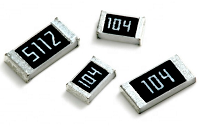## Resistance value marking methods

There are many types of Resistor available in the market which can be used in electrical and electronic circuits to control the flow of current. All resistors have some of "resistive" or "resistance" value. Resistors are available in a range of different resistance values from fractions of an ohm to millions of ohms.

Every resistor has a unique value of resistance, watt, and tolerance value. These values are marked on a resistor in different ways. Few methods are explained below.

### Color Code Method

The resistance value is generally printed onto the body of the resistor as numbers or letters when the resistors body is big enough to read the print, such as large power resistors. But when the resistor is small such as a 1/4 watt carbon or film type, it's difficult to print. For that we are using color bands an alternate to print. So that is called the color code method. The below figure is shown that how the resistance value is marked in 4 bands and 5 bands color code methods.The color order can be remembered by memorize the phrase "B.B. ROY of Great Britain has a Very Good Wife".

### SMD Resistor Code MethodsBecause of the small size of SMD resistors, there is often not space for the color code method to be printed on them. Therefore, new resistor SMD codes were developed. The most commonly seen code methods are the three digit and four-digit code method and an Electronic Industries Alliance (EIA) system called EIA-96.

Three Digit SMD resistor Code Method

A three digit SMT resistor coding method is the one that is normally used for standard tolerance resistors. As the name indicates this SMD resistor marking system uses three digits. The first two digits in the code indicate the significant figures, and the third digit is a multiplier.

##### Example:

The SMD resistor with the digits 103 would have a resistance of 10 x 103 ohms, or 10 kΩ. However, beware of resistors marked with digits such as 470. This is not 470 ohms, but it follows the scheme exactly, and it is 47 x 100 or 47 x 1 = 47 Ω.

If the resistance value is in below 10 Ω, the letter R is used to denote the decimal point. For example, a resistor denoted as 4R7, then the value would be 4.7 Ω.
Four Digit SMD resistor Code Method

The Four digit SMT resistor coding method is the one that is normally used for High tolerance resistors. This SMD resistor marking system uses four digits. The first three digits in the code indicate the significant figures, and the fourth digit is a multiplier.

##### Example:

The SMD resistor with the digits 1002 would have a resistance of 100 x 102 ohms, or 10 kΩ. However, beware of resistors marked with digits such as 4700. This is not 4700 ohms, but it follows the scheme exactly, and it is 470 x 100 or 470 x 1 = 470 Ω.

If the resistance value is in below 100 Ω, the letter R is used to denote the decimal point. For example, a resistor denoted as 47R0, then the value would be 47 Ω.
EIA96 or E-96 SMD resistor Code Method

A further surface mount resistor code scheme has started to be used, and it is aimed at 1% tolerance SMD resistors, i.e. those using the EIA96 or E-96 resistor series. As higher tolerance resistors are used, further figures are needed. However, the small size of SMT resistors makes the figures difficult to read. Accordingly, the new system seeks to address this. Using only three figures, the actual characters can be made larger than those of the four figure system that would otherwise be needed.

The EIA SMD resistor coding scheme uses a three character code: the first 2 numbers indicate the 3 significant digits of the resistor value. The third character is a letter which indicates the multiplier. In this way this SMD resistor marking scheme will not be confused with the 3 figure markings scheme as the letters will differentiate it, although the letter R can be used in both systems. There are only 96 values in the E-96 series, only two figures are needed to number each value, and as a result this is a smart way of reducing the number of characters required.

The table shows EIA SMD resistor code and corresponding value:

CodeValueCodeValueCodeValueCodeValue
01100251784931673562
02102261825032474576
03105271875133275590
04107281915234076604
05110291965334877619
06113302005435778634
07115312055536579649
08118322105637480665
09121332155738381681
10124342215839282698
11127352265940283715
12130362326041284732
13133372376142285750
14137382436243286768
15140392496344287787
16143402556445388806
17147412616546489825
18150422676647590845
19154432746748791866
20158442806849992887
21162452876951193909
22165462947052394931
23169473017153695953
24174483097254996976
CodeMultiplier
Z0.001
Y or R0.01
X or S0.1
A1
B or H10
C100
D1000
E10000
F100000

#### Examples:

01Y = 100 x 0.01 = 1Ω
68X = 499 x 0.1 = 49.9Ω
76X = 604 x 0.1 = 60.4Ω
01A = 100 x 1 = 100Ω
29B = 196 x 10 = 1.96kΩ
01C = 100 x 100 = 10kΩ

### Power rating of the SMD resistor

The Approximate Power Rating Value can be calculated by its dimension. A few commonly used package dimensions with the corresponding typical power ratings are presented in the table below.

Packa­geSize in inches (LxW)Size in mm (LxW)Power rating
02010.024" x 0.012"0.6 mm x 0.3 mm1/20W
04020.04" x 0.02"1.0 mm x 0.5 mm1/16W
06030.063" x 0.031"1.6 mm x 0.8 mm1/16W
08050.08" x 0.05"2.0 mm x 1.25 mm1/10W
12060.126" x 0.063"3.2 mm x 1.6 mm1/8W
12100.126" x 0.10"3.2 mm x 2.5 mm1/4W
18120.18" x 0.12"4.5 mm x 3.2 mm1/3W
20100.20" x 0.10"5.0 mm x 2.5 mm1/2W
25120.25" x 0.12"6.35 mm x 3.2 mm1W
These are estimated power rating only. For accurate power rating value, read the datasheet of SMD resistor.